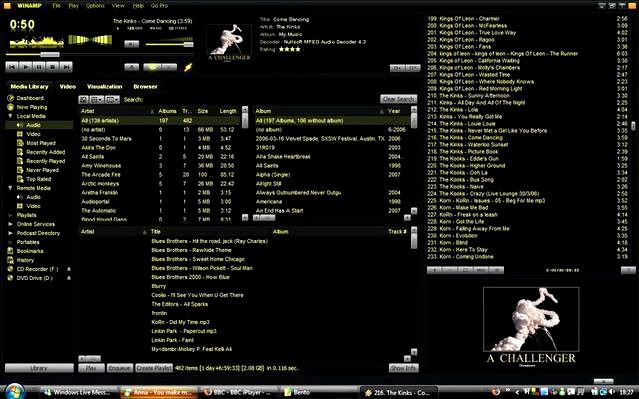View Single Post10th February 2008, 23:56   #147
Josephd
Junior Member

Join Date: Oct 2007
Posts: 2
Hey this is my first attempt at making a colour scheme, I was inspired by " City Night ( neorth mod) " I liked the yellow but I wanted lots more yellow! ( although I think I may of gone slightly over board)

hope at least a few people like it```code:<gammaset id="Night Glow">
<gammagroup id="Titlebar" value="-2915,-2915,-2915" gray="0" boost="0" />
<gammagroup id="TitlebarText" value="0,0,-4046" gray="0" boost="0" />
<gammagroup id="TitlebarMenuText" value="0,0,-4021" gray="0" boost="0" />
<gammagroup id="TitleButtonOrange" value="3291,1281,-1760" gray="0" boost="0" />
<gammagroup id="TitleButtonGrey" value="-453,-453,-428" gray="0" boost="0" />
<gammagroup id="WindowBackground" value="-2664,-2714,-2714" gray="0" boost="0" />
<gammagroup id="WindowText" value="4096,3492,-2664" gray="0" boost="0" />
<gammagroup id="Column Text" value="4096,3015,-2815" gray="0" boost="0" />
<gammagroup id="DisplayText" value="4096,3442,-2413" gray="0" boost="0" />
<gammagroup id="ScrollbarTrack" value="-1835,-1835,-1785" gray="0" boost="0" />
<gammagroup id="PlayerDisplay" value="-4096,-4096,-4096" gray="0" boost="0" />
<gammagroup id="PlayerDisplayGradient" value="-3292,-3318,-3217" gray="0" boost="0" />
<gammagroup id="ComponentFrame" value="-2639,-2689,-2714" gray="0" boost="0" />
<gammagroup id="PlayerButton" value="-1006,-981,-981" gray="0" boost="0" />
<gammagroup id="PlayerShadeSlider" value="-1885,-1885,-1885" gray="0" boost="0" />
<gammagroup id="PlayerButtonPlaceHolder" value="-2513,-2513,-2463" gray="0" boost="0" />
<gammagroup id="PlayerButtonGlow" value="2336,2085,-2363" gray="0" boost="0" />
<gammagroup id="PlayerButtonActive" value="1784,1909,-2262" gray="0" boost="0" />
<gammagroup id="PlayerSliderLight" value="4096,3568,-4096" gray="0" boost="0" />
<gammagroup id="PlayerSliderBackground" value="4096,3166,-2941" gray="0" boost="0" />
<gammagroup id="WindowBackgroundGradient" value="-4046,-4096,-4096" gray="0" boost="0" />
<gammagroup id="CompButtonInactive" value="-2564,-2564,-2539" gray="0" boost="0" />
<gammagroup id="CompButtonActive" value="-2388,-2363,-2363" gray="0" boost="0" />
<gammagroup id="CompButtonInactiveText" value="4096,3266,-2589" gray="0" boost="0" />
<gammagroup id="CompButtonActiveText" value="4096,3266,-2915" gray="0" boost="0" />
<gammagroup id="SelectionBg" value="0,0,-4096" gray="0" boost="0" />
<gammagroup id="SelectionBg Inactive" value="0,0,-1910" gray="0" boost="0" />
<gammagroup id="Selection" value="4096,3518,-4096" gray="0" boost="0" />
<gammagroup id="LightningBolt" value="1457,1507,-1282" gray="0" boost="0" />
<gammagroup id="Column" value="-2714,-2714,-2765" gray="0" boost="0" />
<gammagroup id="ShadeButton" value="0,0,-3795" gray="0" boost="0" />
<gammagroup id="NotifierBackground" value="-2639,-2639,-2639" gray="0" boost="0" />
<gammagroup id="NotifierText" value="4096,3266,-2714" gray="0" boost="0" />
<gammagroup id="NotifierTextShadow" value="0,0,0" gray="0" boost="0" />
<gammagroup id="NotifierUpperTextShadow" value="0,0,0" gray="0" boost="0" />
<gammagroup id="NotifierUpperText" value="4096,3417,-2539" gray="0" boost="0" />
<gammagroup id="Frame_Borders" value="-2312,-2388,-2337" gray="0" boost="0" />
</gammaset>```

please excuse my desktop on the print screen forgot to crop

Oh also I used paint to save my print screen and thusly the image has lost some colour intensity ( the yellow is actually very yellow )Attached Filesnight glow.xml (3.2 KB, 1077 views)